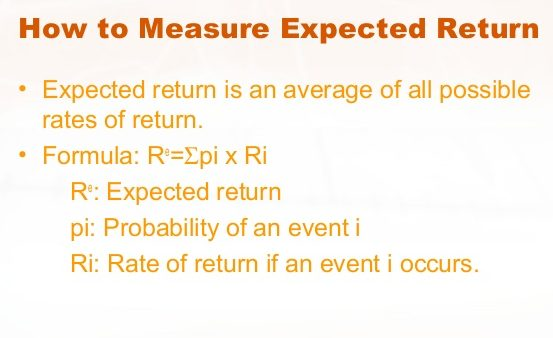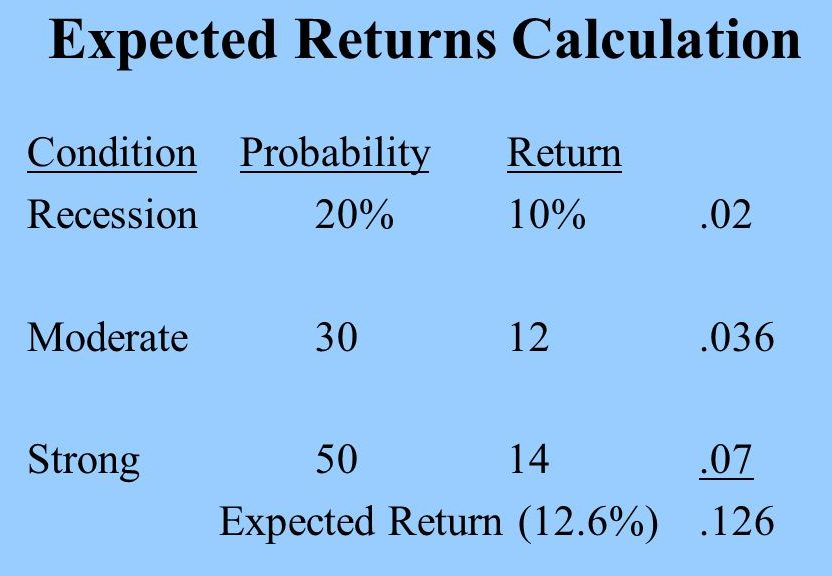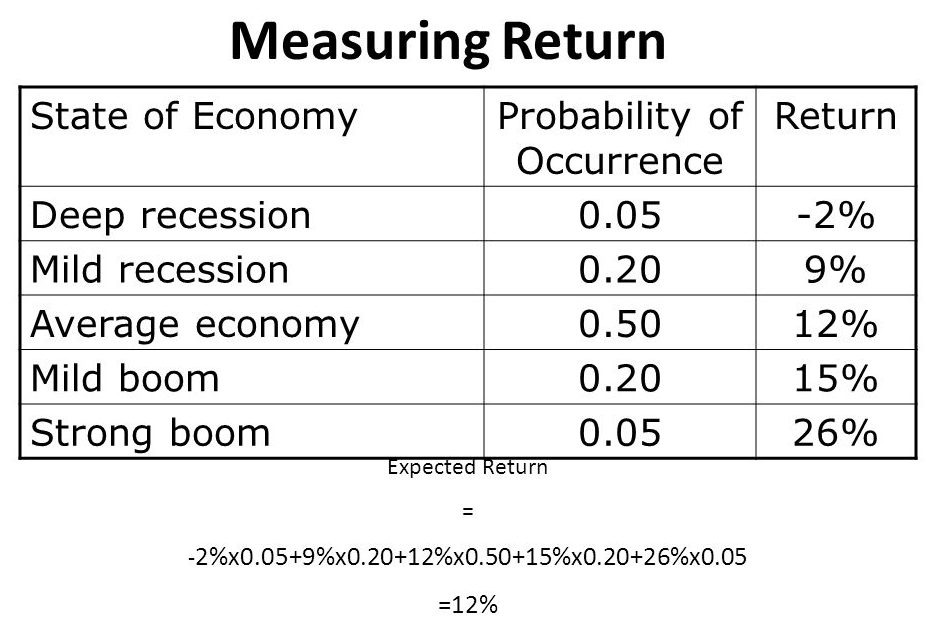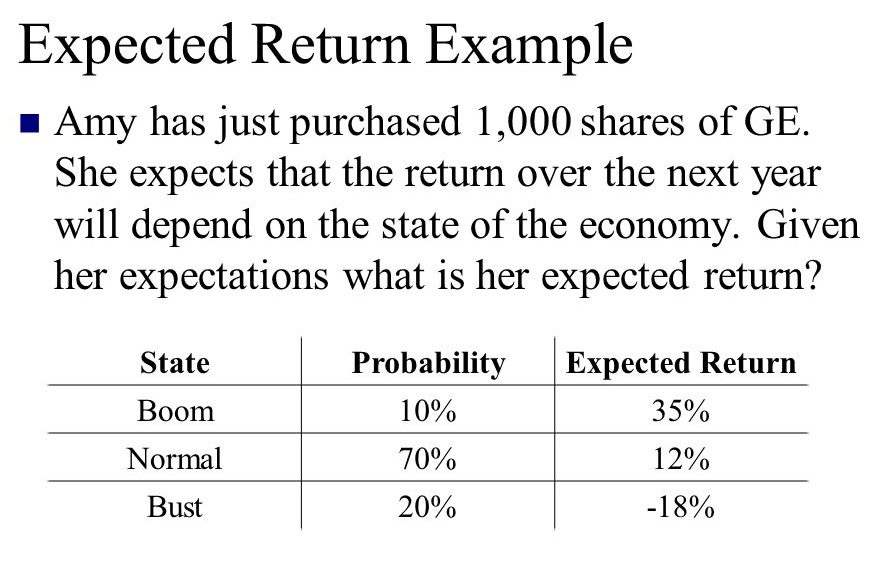Latest Articles
Home / Finance / Corporate Finance / Measuring Expected Returns

# Measuring Expected Returns

Measuring Expected Returns. Returns from security consist of income in the form of dividend or interest plus change in capital. Total returns consist of all price changes and income received during a specific interval. Future returns are the meaningful ones for decision made today. An important use of expected return is to compare value across asset classes and across time. Some asset allocation decisions are based on the risk premium differential, which is the difference between the expected return on assets such as stocks, bonds, or real estate and the expected return on a risk-free asset such as a Treasury bill.

An important way to assess return is to compare historical (ex post) and expected (ex ante) returns. Historical returns are generated by the history of the performance of an investment over a specified time period. Expected returns, on the other hand, are the best estimates of what returns might be over some future time period. Historical returns are known with certainty whereas expected returns are fraught with uncertainty i.e., they are probabilistic in nature.

### Measures of Expected Returns

Investments are made to generate income, to appreciate capital and to preserve capital. An investment periodically generates cash for the investor in the form of interest, dividends, or rent. The market price or the value of an investment may rise or fall over time. A capital gain arises when the market value of an asset is above the price what is paid to acquire the asset. Capital loss arises when the market price fall below what is paid for the asset. In order to measure the return generated by an investment, one should account for both the income generated by the investment during the period the asset was held and the change in the price. Investors in common stock consider the change in price and the amount of dividends declared by the company during the holding period of the asset.

For bonds, the holding period return is equal to the price change and interest received. Investors are concerned to measure the return and choose among alternative investment assets. To meet this, the investors are likely to measure both historical (ex post) and expected (ex ante) rates of return. Historical returns are often used by the investors with a view to estimating the expected rates of return. The first measure of return from an individual investment is the historical rate of return during the period the investment is held. The second measure of return is the measure of expected rate of return for an investment.

The period during which an investor owns an asset is termed as its holding period and the percentage return from the investment and price changes during this period is called holding period return. For a perpetual security like stock, the holding period return for a particular period of time is equal to the sum of the price change plus dividends received for that period divided by the price at the beginning of the time period.

For fixed income security like bond, the holding period return is equal to the price change plus interest received divided by the beginning price.

However, in general, the holding period return is calculated as follows –

rit = [(Pit -Pi,t1) + Dit]/ Pi,t1

where,

rit  = Return for stock i at time t,

Pit = Price of the stock i at time t,

Pi,t1 = Price of the stock i at time t-1 and

Dit = Dividends declared for stock i at time t

To measure the return generated by an investment, the price change and the cash flow derived from the investment during the period must be taken into account.###~ Measuring Expected Returns ~